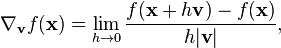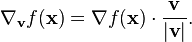# Gradient of a scalar function

• I
Hi, I am looking for a proof that explains why gradient is a vector that points to the greatest increase of a scalar function at a given point p.

http://math.stackexchange.com/quest...always-be-directed-in-an-increasing-direction

I understand the proof here. But.. the idea here is del(f)*dl = df is maximized when del(f) and dl point to the same direction, and that maximizes df. Then we have to first consider the direction of dl to verify where del(f) points to.

If we assume that there is a multivariable function f(x1, x2, x3, . . . xn)
and lets say that the derivative with respect to xj is a negative value at p0.
(also derivatives with respect to other variable x1, x2, x3 . . . xn are positive)

which indicates that the peak is at the left of the graph (at the negative direction with respect to point p0)
then, del(f)*dl = df will be maximized when df/dxj*(-dxj) because it would give positive incremental df, since the derivative is negative at p0.

also, we can do this because dl is a vector quantity so we can define its direction as we want it to be.
But, total differential doesnt take this into account. It just multiplies a small increment of each variable, dxi. and they all have the same sign.

I think this is a contradiction.

Twigg
Gold Member
I think what's confusing you is the use of ## dx_{j}##. In this context, it doesn't mean a small positive infinitesimal quantity, as it would in the context of integrating over an area, as in ##dA = dx dy##. In this context, ##dx_{j}## is meant to represent a displacement (be it positive or negative) in the ##x_{j}## coordinate. The author of the post you linked likely uses this notation because it agrees with the theory of differential forms. This works if you always remember that the dx's aren't the fundamental changes and treat them instead as parametric differentials. In other words, let ## (c_{1}, . . ., c_{n}) ## be the point you want to take the gradient of ## f(x_{1},...,x_{n}) ## (which must be smooth and non-critical at the point ##c##). Consider an arbitrary smooth regular curve through ##\vec{c}## given by ## \gamma (\epsilon) ## defined over a closed interval ##-a \leq \epsilon \leq +a ## where ##\gamma(0) = \vec{c}##. For an infinitesimally small increment ##d\epsilon## in the curve parameter ##\epsilon##, the displacement of the point ##\vec{c}## along the curve is given by the differential ##d\vec{\mathcal{l}} = \sum_{i} \dot{\gamma_{i}}(0) \vec{e}_{i} d\epsilon##. In that sense, each component differential ##dx{i} = \dot{\gamma_{i}}(0) \vec{e}_{i} d\epsilon## can be either positive or negative depending on the velocity of the curve ##\gamma## as it passes through point ##\vec{c}##.

I think what's confusing you is the use of ## dx_{j}##. In this context, it doesn't mean a small positive infinitesimal quantity, as it would in the context of integrating over an area, as in ##dA = dx dy##. In this context, ##dx_{j}## is meant to represent a displacement (be it positive or negative) in the ##x_{j}## coordinate. The author of the post you linked likely uses this notation because it agrees with the theory of differential forms. This works if you always remember that the dx's aren't the fundamental changes and treat them instead as parametric differentials. In other words, let ## (c_{1}, . . ., c_{n}) ## be the point you want to take the gradient of ## f(x_{1},...,x_{n}) ## (which must be smooth and non-critical at the point ##c##). Consider an arbitrary smooth regular curve through ##\vec{c}## given by ## \gamma (\epsilon) ## defined over a closed interval ##-a \leq \epsilon \leq +a ## where ##\gamma(0) = \vec{c}##. For an infinitesimally small increment ##d\epsilon## in the curve parameter ##\epsilon##, the displacement of the point ##\vec{c}## along the curve is given by the differential ##d\vec{\mathcal{l}} = \sum_{i} \dot{\gamma_{i}}(0) \vec{e}_{i} d\epsilon##. In that sense, each component differential ##dx{i} = \dot{\gamma_{i}}(0) \vec{e}_{i} d\epsilon## can be either positive or negative depending on the velocity of the curve ##\gamma## as it passes through point ##\vec{c}##.

But it seems still unclear to me somehow. well, i maybe asking you stupid questions but everything gets suspicious when I think too much of something.

1.
I thought that each incremental dxi should be all positive or all negative because that would give maximum df.It is obvious that incremental df is dependent on the direction of increment hv at a given point p0.
if we look at the graph of f vs xj, because the derivative is negative, it should increase to the negative direction.
For each coordinate x1 to xn, we are free to choose the direction(either negative or positive) to increase the total differential sum of dl.
But the differential dxi has to have the same sign to maximize dl.

2.

Do you mean the parametrized differential dx by dx = lim(eps->0)|eps|?